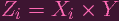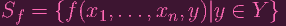I.3.15ac_ERRATA

5/15/2021

Ahhhh, the smell of errata in the morning. 'Tis been a while since I've casually stated a false statement and not gotten away with it. Let's take a look.

SinceZi is clearly generated by polynomials that have no Y terms, and these polynomials determine Xi.

Firstly, I meant to say "no yi terms". There is no such thing as a "Y " term, since Y is a variety, not an indeterminate in a polynomial ring. Secondly, that would still be wrong. Let's try this again.

Let f I(Zi). Define(i.e. analogous to before, plug in y for the yi coordinates and leave the xi coordinates as indeterminates, giving us a polynomial in k[{xi}]. We do this for every y Y ). Then

 x ∈ Xi ⇐⇒x × Y ⊂ Xi × Y ⇐⇒∀y ∈ Y : x × y ∈ Zi ⇐⇒∀y ∈ Y,∀f ∈ I(Zi) : f(x × y) = 0 ⇐⇒∀f ∈ I(Zi),∀y ∈ Y : f(x × y) = 0 ⇐⇒∀f ∈ I(Zi),∀g ∈ Sf : g(x) = 0 ⇐⇒∀g ∈⋃ f∈I(Zi)Sf : g(x) = 0 ⇐⇒x ∈ Z(⋃ f∈I(Zi)Sf)

That last set is closed, so Xi is closed. So that's fixed.

Also, boy, I am slowing down in updates again. The ebb and flow of Hartshorned is mysterious, dependent more on your dear admin's whim than their actual busyness. A new week is upon us, let's see how we do.